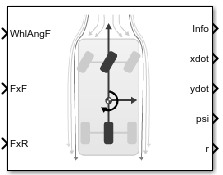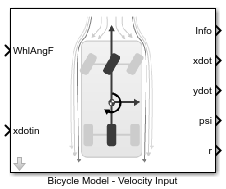# Bicycle Model

Implement a single track 3DOF rigid vehicle body to calculate longitudinal, lateral, and yaw motion

•Libraries:
Automated Driving Toolbox / Driving Scenario and Sensor Modeling
Vehicle Dynamics Blockset / Vehicle Body

## Description

The Bicycle Model block implements a rigid two-axle single track vehicle body model to calculate longitudinal, lateral, and yaw motion. The block accounts for body mass, aerodynamic drag, and weight distribution between the axles due to acceleration and steering. There are two types of Bicycle Model blocks.

BlockImplementation

Bicycle Model - Velocity Input• Block assumes that the external longitudinal velocity is quasi-steady state so the longitudinal acceleration is approximately zero.

• Since the motion is quasi-steady, the block calculates only lateral forces using the tire slip angles and linear cornering stiffness.

Bicycle Model - Force Input• Block uses the external longitudinal force to accelerate or brake the vehicle.

• Block calculates lateral forces using the tire slip angles and linear cornering stiffness.

To calculate the normal forces on the front and rear axles, the block uses rigid-body vehicle motion, suspension system forces, and wind and drag forces. The block resolves the force and moment components on the rigid vehicle body frame.

## Ports

### Input

expand all

Longitudinal force on the front axle, FxF, along vehicle-fixed `x`-axis, in N.

Bicycle Model - Force Input block input port.

Longitudinal force on the rear axle, FxR, along vehicle-fixed `x`-axis, in N.

Bicycle Model - Force Input block input port.

Vehicle CG velocity along vehicle-fixed x-axis, in m/s.

Bicycle Model - Velocity Input block input port.

### Output

expand all

Bus signal containing these block values.

SignalDescriptionValueUnits
`InertFrm``Cg``Disp``X`Vehicle CG displacement along the earth-fixed X-axis

Computed

m
`Y`Vehicle CG displacement along the earth-fixed Y-axis

Computed

m

`Z`Vehicle CG displacement along the earth-fixed Z-axis`0`m
`Vel``Xdot`Vehicle CG velocity along the earth-fixed X-axis

Computed

m/s

`Ydot`Vehicle CG velocity along the earth-fixed Y-axis

Computed

m/s
`Zdot`Vehicle CG velocity along the earth-fixed Z-axis`0`m/s
`Ang``phi`Rotation of the vehicle-fixed frame about the earth-fixed X-axis (roll)`0`rad
`theta`Rotation of the vehicle-fixed frame about the earth-fixed Y-axis (pitch)`0`rad
`psi`Rotation of the vehicle-fixed frame about the earth-fixed Z-axis (yaw)

Computed

`FrntAxl``Disp``X`Front wheel displacement along the earth-fixed X-axis

Computed

m
`Y`Front wheel displacement along the earth-fixed Y-axis

Computed

m
`Z`Front wheel displacement along the earth-fixed Z-axis`0`m
`Vel``Xdot`Front wheel velocity along the earth-fixed X-axis

Computed

m/s
`Ydot`Front wheel velocity along the earth-fixed Y-axis

Computed

m/s
`Zdot`Front wheel velocity along the earth-fixed Z-axis`0`m/s
`RearAxl``Disp``X`Rear wheel displacement along the earth-fixed X-axis

Computed

m
`Y`Rear wheel displacement along the earth-fixed Y-axis

Computed

m
`Z`Rear wheel displacement along the earth-fixed Z-axis`0`m
`Vel``Xdot`Rear wheel velocity along the earth-fixed X-axis

Computed

m/s
`Ydot`Rear wheel velocity along the earth-fixed Y-axis

Computed

m/s
`Zdot`Rear wheel velocity along the earth-fixed Z-axis`0`m/s
`Hitch``Disp``X`Hitch offset from axle plane along the earth-fixed X-axis

Computed

m
`Y`Hitch offset from center plane along the earth-fixed Y-axis

Computed

m
`Z`Hitch offset from axle plane along the earth-fixed Z-axis

Computed

m
`Vel``Xdot`Hitch offset velocity from axle plane along the earth-fixed X-axis

Computed

m
`Ydot`Hitch offset velocity from center plane along the earth-fixed Y-axis

Computed

m
`Zdot`Hitch offset velocity from axle plane along the earth-fixed Z-axis

Computed

m
`Geom``Disp``X`Vehicle chassis offset from axle plane along the earth-fixed X-axis

Computed

m
`Y`Vehicle chassis offset from center plane along the earth-fixed Y-axis

Computed

m
`Z`Vehicle chassis offset from axle plane along the earth-fixed Z-axis

Computed

m
`Vel``Xdot`Vehicle chassis offset velocity along the earth-fixed X-axis

Computed

m/s
`Ydot`Vehicle chassis offset velocity along the earth-fixed Y-axis

Computed

m/s
`Zdot`Vehicle chassis offset velocity along the earth-fixed Z-axis

Computed

m/s
`BdyFrm``Cg``Vel``xdot`Vehicle CG velocity along the vehicle-fixed x-axis

Computed

m/s
`ydot`Vehicle CG velocity along the vehicle-fixed y-axis

Computed

m/s
`zdot`Vehicle CG velocity along the vehicle-fixed z-axis`0`m/s
`Ang``Beta`

Body slip angle, β

`$\beta =\frac{{V}_{y}}{{V}_{x}}$`

Computed

`AngVel``p`Vehicle angular velocity about the vehicle-fixed x-axis (roll rate)`0`rad/s
`q`Vehicle angular velocity about the vehicle-fixed y-axis (pitch rate)`0`rad/s
`r`Vehicle angular velocity about the vehicle-fixed z-axis (yaw rate)

Computed

`Acc``ax`Vehicle CG acceleration along the vehicle-fixed x-axis

Computed

gn
`ay`Vehicle CG acceleration along the vehicle-fixed y-axis

Computed

gn
`az`Vehicle CG acceleration along the vehicle-fixed z-axis`0`gn
`xddot`Vehicle CG acceleration along the vehicle-fixed x-axis

Computed

m/s^2
`yddot`Vehicle CG acceleration along the vehicle-fixed y-axis

Computed

m/s^2
`zddot`Vehicle CG acceleration along the vehicle-fixed z-axis`0`m/s^2
`AngAcc``pdot`Vehicle angular acceleration about the vehicle-fixed x-axis`0`rad/s
`qdot`Vehicle angular acceleration about the vehicle-fixed y-axis`0`rad/s
`rdot`Vehicle angular acceleration about the vehicle-fixed z-axis

Computed

`DCM`

Direction cosine matrix

Computed

`Forces``Body``Fx`Net force on vehicle CG along the vehicle-fixed x-axis

Computed

N
`Fy`Net force on vehicle CG along the vehicle-fixed y-axis

Computed

N
`Fz`Net force on vehicle CG along the vehicle-fixed z-axis`0`N
`Ext``Fx`External force on vehicle CG along the vehicle-fixed x-axis

Computed

N
`Fy`External force on vehicle CG along the vehicle-fixed y-axis

Computed

N
`Fz`External force on vehicle CG along the vehicle-fixed z-axis`0`N
`Hitch``Fx`

Hitch force applied to body at the hitch location along the vehicle-fixed x-axis

Input

N
`Fy`

Hitch force applied to body at the hitch location along the vehicle-fixed y-axis

Input

N
`Fz`

Hitch force applied to body at the hitch location along the vehicle-fixed z-axis

Input

N
`FrntAxl``Fx`

Longitudinal force on front wheel, along the vehicle-fixed x-axis

Computed

N
`Fy`

Lateral force on front wheel along the vehicle-fixed y-axis

Computed

N
`Fz`

Normal force on front wheel, along the vehicle-fixed z-axis

Computed

N
`RearAxl``Fx`

Longitudinal force on rear wheel, along the vehicle-fixed x-axis

Computed

N
`Fy`

Lateral force on rear wheel along the vehicle-fixed y-axis

Computed

N
`Fz`

Normal force on rear wheel, along the vehicle-fixed z-axis

ComputedN
`Tires``FrntTire``Fx`

Front tire force, along the vehicle-fixed x-axis

ComputedN
`Fy`

Front tire force, along the vehicle-fixed y-axis

ComputedN
`Fz`

Front tire force, along the vehicle-fixed z-axis

ComputedN
`RearTire``Fx``Fx`

Rear tire force, along the vehicle-fixed x-axis

ComputedN
`Fy`

Rear tire force, along the vehicle-fixed y-axis

ComputedN
`Fz`

Rear tire force, along the vehicle-fixed z-axis

ComputedN
`Drag``Fx`Drag force on vehicle CG along the vehicle-fixed x-axis

Computed

N
`Fy`Drag force on vehicle CG along the vehicle-fixed y-axis

Computed

N
`Fz`Drag force on vehicle CG along the vehicle-fixed z-axis

Computed

N
`Grvty``Fx`Gravity force on vehicle CG along the vehicle-fixed x-axis

Computed

N
`Fy`Gravity force on vehicle CG along the vehicle-fixed y-axis

Computed

N
`Fz`Gravity force on vehicle CG along the vehicle-fixed z-axis

Computed

N
`Moments``Body``Mx`Body moment on vehicle CG about the vehicle-fixed x-axis`0`N·m
`My`Body moment on vehicle CG about the vehicle-fixed y-axis

Computed

N·m
`Mz`Body moment on vehicle CG about the vehicle-fixed z-axis`0`N·m
`Drag``Mx`Drag moment on vehicle CG about the vehicle-fixed x-axis`0`N·m
`My`Drag moment on vehicle CG about the vehicle-fixed y-axis

Computed

N·m
`Mz`Drag moment on vehicle CG about the vehicle-fixed z-axis`0`N·m
`Ext``Mx`External moment on vehicle CG about the vehicle-fixed x-axis`0`N·m
`My`External moment on vehicle CG about the vehicle-fixed y-axis

Computed

N·m
`Mz`External moment on vehicle CG about the vehicle-fixed z-axis`0`N·m
`Hitch``Mx`Hitch moment at the hitch location about vehicle-fixed x-axis`0`N·m
`My`Hitch moment at the hitch location about vehicle-fixed y-axis

Computed

N·m
`Mz`Hitch moment at the hitch location about vehicle-fixed z-axis`0`N·m
`FrntAxl``Disp``x`Front wheel displacement along the vehicle-fixed x-axis

Computed

m
`y`Front wheel displacement along the vehicle-fixed y-axisComputedm
`z`Front wheel displacement along the vehicle-fixed z-axis

Computed

m
`Vel``xdot`Front wheel velocity along the vehicle-fixed x-axis

Computed

m/s
`ydot`Front wheel velocity along the vehicle-fixed y-axis

Computed

m/s
`zdot`Front wheel velocity along the vehicle-fixed z-axis`0`m/s
`Steer``WhlAngFL`

Front left wheel steering angle

Computed

`WhlAngFR`

Front right wheel steering angle

Computed

`RearAxl``Disp``x`Rear wheel displacement along the vehicle-fixed x-axis

Computed

m
`y`Rear wheel displacement along the vehicle-fixed y-axisComputedm
`z`Rear wheel displacement along the vehicle-fixed z-axis

Computed

m
`Vel``xdot`Rear wheel velocity along the vehicle-fixed x-axis

Computed

m/s
`ydot`Rear wheel velocity along the vehicle-fixed y-axis

Computed

m/s
`zdot`Rear wheel velocity along the vehicle-fixed z-axis`0`m/s
`Steer``WhlAngRL`

Rear left wheel steering angle

Computed

`WhlAngRR`

Rear right wheel steering angle

Computed

`Hitch``Disp``x`Hitch offset from axle plane along the vehicle-fixed x-axis

Input

m
`y`Hitch offset from center plane along the vehicle-fixed y-axis

Input

m
`z`Hitch offset from axle plane along the earth-fixed z-axis

Input

m
`Vel``xdot`Hitch offset velocity along the vehicle-fixed x-axis

Computed

m/s
`ydot`Hitch offset velocity along the vehicle-fixed y-axis

Computed

m/s
`zdot`Hitch offset velocity along the vehicle-fixed z-axis

Computed

m/s
`Pwr``Ext`Applied external power

Computed

W
`Hitch`Power loss due to hitch

Computed

W
`Drag`Power loss due to drag

Computed

W
`Geom``Disp``x`Vehicle chassis offset from axle plane along the vehicle-fixed x-axis

Input

m
`y`Vehicle chassis offset from center plane along the vehicle-fixed y-axis

Input

m
`z`Vehicle chassis offset from axle plane along the earth-fixed z-axis

Input

m
`Vel``xdot`Vehicle chassis offset velocity along the vehicle-fixed x-axis

Computed

m/s
`ydot`Vehicle chassis offset velocity along the vehicle-fixed y-axis

Computed

m/s
`zdot`Vehicle chassis offset velocity along the vehicle-fixed z-axis`0`m/s
`Ang``Beta`

Body slip angle, β

`$\beta =\frac{{V}_{y}}{{V}_{x}}$`

Computed

SignalDescriptionValueUnits
`PwrInfo``PwrTrnsfrd``PwrFxExt`Externally applied longitudinal force power

Computed

W
`PwrFyExt`Externally applied lateral force power

Computed

W
`PwrMzExt`Externally applied roll moment power

Computed

W
`PwrFwFx`Longitudinal force applied at the front axle power

Computed

W
`PwrFwFy`Lateral force applied at the front axle power

Computed

W
`PwrFwRx`Longitudinal force applied at the rear axle power

Computed

W
`PwrFwRy`Lateral force applied at the rear axle power

Computed

W
`PwrNotTrnsfrd``PwrFxDrag`Longitudinal drag force power

Computed

W
`PwrFyDrag`Lateral drag force power

Computed

W
`PwrMzDrag`Drag pitch moment power

Computed

W
`PwrStored``PwrStoredGrvty`Rate change in gravitational potential energy

Computed

W
`PwrStoredxdot`Rate of change of longitudinal kinetic energy

Computed

W
`PwrStoredydot`Rate of change of lateral kinetic energy

Computed

W
`PwrStoredr`Rate of change of rotational yaw kinetic energy

Computed

W

Vehicle CG velocity along vehicle-fixed `x`-axis, in m/s.

Vehicle CG velocity along vehicle-fixed `y`-axis, in m/s.

Rotation of the vehicle-fixed frame about earth-fixed `Z`-axis (yaw), in rad..

Vehicle angular velocity, `r`, about the vehicle-fixed `z`-axis (yaw rate), in rad/s.

## Parameters

expand all

Longitudinal

Number of wheels on front axle, NF. The value is dimensionless.

Number of wheels on rear axle, NR. The value is dimensionless.

Vehicle mass, m, in kg.

Horizontal distance a from the vehicle CG to the front wheel axle, in m.

Horizontal distance b from the vehicle CG to the rear wheel axle, in m.

Height of vehicle CG above the axles, h, in m.

Initial vehicle CG displacement along earth-fixed X-axis, in m.

Initial vehicle CG velocity along vehicle-fixed x-axis, in m/s.

Lateral

Front tire corner stiffness, Cyf, in N/rad.

Rear tire corner stiffness, Cyr, in N/rad.

Initial vehicle CG displacement along earth-fixed Y-axis, in m.

Initial vehicle CG velocity along vehicle-fixed y-axis, in m/s.

Yaw

Yaw polar inertia, in kg*m^2.

Aerodynamic

Effective vehicle cross-sectional area, Af, to calculate the aerodynamic drag force on the vehicle, in m2.

Air drag coefficient, Cd. The value is dimensionless.

Air lift coefficient, Cl. The value is dimensionless.

Longitudinal drag pitch moment coefficient, Cpm. The value is dimensionless.

Relative wind angle vector, βw, in rad.

Side force coefficient vector coefficient, Cs. The value is dimensionless.

Yaw moment coefficient vector, Cym. The value is dimensionless.

Environment

Environmental absolute pressure, Pabs, in Pa.

Environmental absolute temperature, T, in K.

Gravitational acceleration, g, in m/s^2.

Nominal friction scale factor, μ. The value is dimensionless.

Simulation

Longitudinal velocity tolerance, in m/s.

Nominal normal force, in N.

Vehicle chassis offset from axle plane along body-fixed x-axis, in m. When you use the 3D visualization engine, consider using the offset to locate the chassis independent of the vehicle CG.

Vehicle chassis offset from center plane along body-fixed y-axis, in m. When you use the 3D visualization engine, consider using the offset to locate the chassis independent of the vehicle CG.

Vehicle chassis offset from axle plane along body-fixed z-axis, in m. When you use the 3D visualization engine, consider using the offset to locate the chassis independent of the vehicle CG.

 Gillespie, Thomas. Fundamentals of Vehicle Dynamics. Warrendale, PA: Society of Automotive Engineers (SAE), 1992.